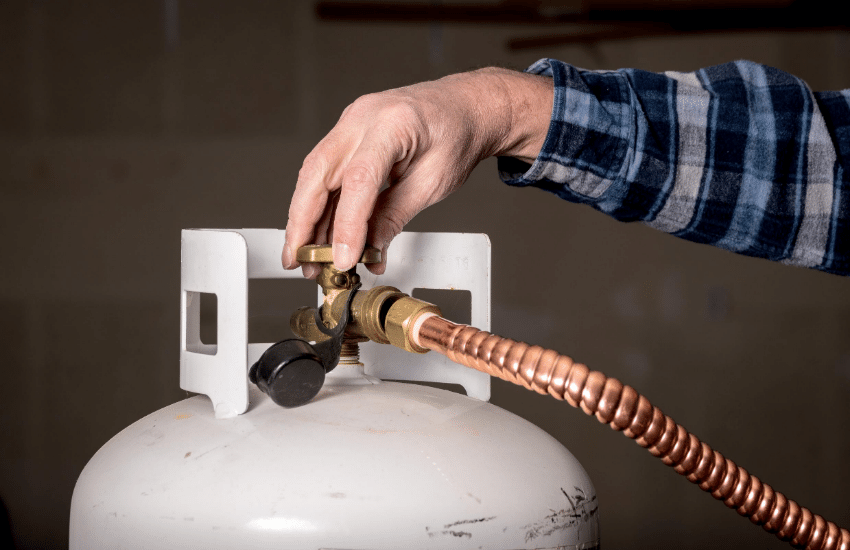# How Many Gallons of Propane in a 20, 30, 40, and 100-pound tanks?There are 0.236 gallons in one pound of propane. Therefore, in a 20-pound propane tank. there are 4.72 gallons of propane (20 lbs x 0.236 gallons per lb = 4.72 gallons).

The table below provides the gallons of propane in common propane tank sizes. If your propane tank size is not listed below, simply multiply the tank size in pounds by 0.236 to determine the gallons of propane in a given tank.